Next: Ideal Amplifier Approximation Up: Operational Amplifiers Previous: Operational Amplifiers

# Open-Loop Amplifiers

Figure 6.1a shows a complete diagram of an operational amplifier. A more common version of the diagram is shown in figure 6.1b, where missing parts are assumed to exist. The inverting input means that the output signal will be 180out of phase with the input applied to this terminal. On the diagram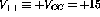V (DC) and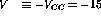V (DC).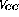is typically, but not necessarily,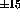V. The positive and negative voltages are necessary to allow the amplification of both positive and negative signals without special biasing.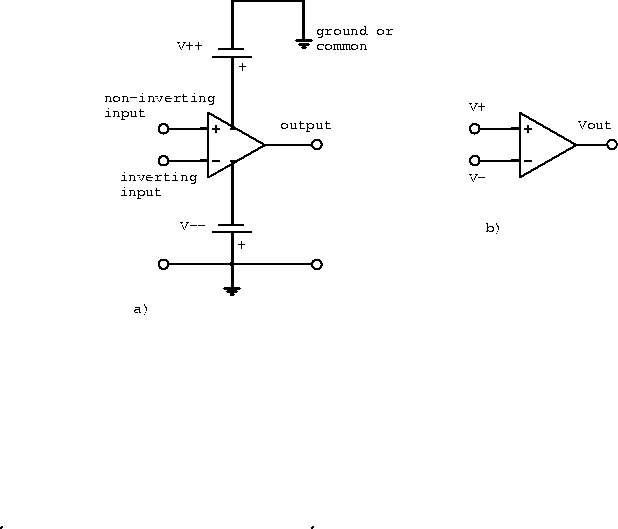Figure 6.1:  a) Complete diagram of an operational amplifier and b) common diagram of an operational amplifier.

For a linear amplifier (cf. a differential amplifier) the open-loop gain is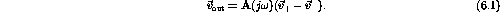The open-loop gain can be approximated by the transfer function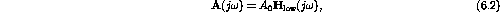where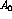is the DC open-loop gain and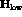is the transfer function of a passive low-pass filter. We can write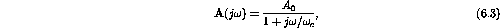where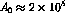and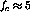Hz.

Two conditions must be satisfied for linear operation:

1. The input voltage must operate within the bias voltages: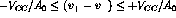.
2. For no clipping the output voltage swing must be restricted to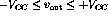.

Doug Gingrich
Tue Jul 13 16:55:15 EDT 1999